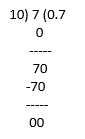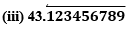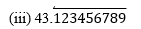NCERT solutions Class 10 Maths Chapter 1 Real Numbers Exercise 1.4 can be found here. We have our own subject experts to prepare these NCERT Maths Solutions for Class 10. They also review these solutions from time to time to modify them to make it easily understandable by the students.

These solutions can be practised easily and help the students to revise it quickly. This exercise 1.4 which is the fourth exercise of chapter 1 Real numbers in class 10 explains Rational numbers and its decimal expansion in further detail. To make it easy to grasp, the solutions are given in a stepwise method. Not only this, we have also focused on following all the NCERT guidelines while preparing the solutions.

### Access Answers of Maths NCERT Class 10 Chapter 1 – Real Number Exercise 1.4 page number 7

Exercise 1.1 Solutions 5 Question ( 4 long, 1 short)
Exercise 1.2 Solutions 7 Question ( 4 long, 3 short)
Exercise 1.3 Solutions 3 Question ( 3 short)

1. Without actually performing the long division, state whether the following rational numbers will have a terminating decimal expansion or a non-terminating repeating decimal expansion:

(i) 13/3125 (ii) 17/8 (iii) 64/455 (iv) 15/1600 (v) 29/343 (vi) 23/(2352) (vii) 129/(225775) (viii) 6/15 (ix) 35/50 (x) 77/210

Solutions:

Note: If the denominator has only factors of 2 and 5 or in the form of 2m ×5n then it has terminating decimal expansion.

If the denominator has factors other than 2 and 5 then it has a non-terminating decimal expansion.

(i) 13/3125

Factorizing the denominator, we get,

3125 = 5 × 5 × 5 × 5 × 5 = 55

Since, the denominator has only 5 as its factor, 13/3125 has a terminating decimal expansion.

(ii) 17/8

Factorizing the denominator, we get,

8 = 2×2×2 = 23

Since, the denominator has only 2 as its factor, 17/8 has a terminating decimal expansion.

(iii) 64/455

Factorizing the denominator, we get,

455 = 5×7×13

Since, the denominator is not in the form of 2m × 5n, thus 64/455 has a non-terminating decimal expansion.

(iv) 15/ 1600

Factorizing the denominator, we get,

1600 = 26×52

Since, the denominator is in the form of 2m × 5n, thus 15/1600 has a terminating decimal expansion.

(v) 29/343

Factorizing the denominator, we get,

343 = 7×7×7 = 73 Since, the denominator is not in the form of 2m × 5n thus 29/343 has a non-terminating decimal expansion.

(vi)23/(2352)

Clearly, the denominator is in the form of 2m × 5n.

Hence, 23/ (2352) has a terminating decimal expansion.

(vii) 129/(225775)

As you can see, the denominator is not in the form of 2m × 5n.

Hence, 129/ (225775) has a non-terminating decimal expansion.

(viii) 6/15

6/15 = 2/5

Since, the denominator has only 5 as its factor, thus, 6/15 has a terminating decimal expansion.

(ix) 35/50

35/50 = 7/10

Factorising the denominator, we get,

10 = 2 × 5

Since, the denominator is in the form of 2m × 5n thus, 35/50 has a terminating decimal expansion.

(x) 77/210

77/210 = (7× 11)/ (30 × 7) = 11/30

Factorising the denominator, we get,

30 = 2 × 3 × 5

As you can see, the denominator is not in the form of 2m × 5n .Hence, 77/210 has a non-terminating decimal expansion.

2. Write down the decimal expansions of those rational numbers in Question 1 above which have terminating decimal expansions.

Solutions:

(i) 13/312513/3125 = 0.00416

(ii) 17/817/8 = 2.125

(iii) 64/455 has a Non terminating decimal expansion

(iv)15/ 160015/1600 = 0.009375

(v) 29/ 343 has a Non terminating decimal expansion

(vi)23/ (2352) = 23/(8×25)= 23/20023/ (2352) = 0.115

(vii) 129/ (225775) has a Non terminating decimal expansion

(viii) 6/15 = 2/5(ix) 35/50 = 7/1035/50 = 0.7

(x) 77/210 has a non-terminating decimal expansion.

3. The following real numbers have decimal expansions as given below. In each case, decide whether they are rational or not. If they are rational, and of the form, p q what can you say about the prime factors of q?

(i) 43.123456789

(ii) 0.120120012000120000. . .Solutions:

(i) 43.123456789

Since it has a terminating decimal expansion, it is a rational number in the form of p/q and q has factors of 2 and 5 only.

(ii) 0.120120012000120000. . .

Since, it has non-terminating and non- repeating decimal expansion, it is an irrational number.Since it has non-terminating but repeating decimal expansion, it is a rational number in the form of p/q and q has factors other than 2 and 5.

NCERT solutions for class 10 maths chapter 1 – Real Numbers Exercise 1.4 is the fourth exercise of Chapter 1 of class 10 Maths. After introducing Real Numbers in Class 9, it is discussed in further detail in Class 10. In this class, It explains the rational numbers and their decimal expansions. The exercise discusses when exactly the decimal expansion of a rational number is terminating and when it is non-terminating.

• Revisiting Rational Numbers and their decimal expansions – It includes 3 questions where question 1 has 10 parts and question 3 has three parts.

### Key Features of NCERT Solutions for Class 10 Maths Chapter 1- Real Number Exercise 1.4

• These NCERT Solutions help you solve and revise all questions of Exercise 1.4.
• You’ll be able to solve the problems more confidently after going through these NCERT Maths Solutions.
• It not only helps in revising the concept but also makes you score well in exams.
• It follows NCERT guidelines which help in preparing the students accordingly.
• It contains all the important questions from the examination point of view.